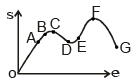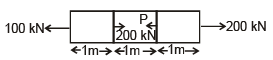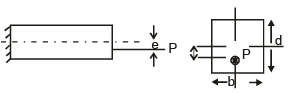Test: Strength of Materials - 1

# Test: Strength of Materials - 1 - Mechanical Engineering

Test Description

## 25 Questions MCQ Test Mechanical Engineering SSC JE (Technical) - Test: Strength of Materials - 1

Test: Strength of Materials - 1 for Mechanical Engineering 2023 is part of Mechanical Engineering SSC JE (Technical) preparation. The Test: Strength of Materials - 1 questions and answers have been prepared according to the Mechanical Engineering exam syllabus.The Test: Strength of Materials - 1 MCQs are made for Mechanical Engineering 2023 Exam. Find important definitions, questions, notes, meanings, examples, exercises, MCQs and online tests for Test: Strength of Materials - 1 below.
Solutions of Test: Strength of Materials - 1 questions in English are available as part of our Mechanical Engineering SSC JE (Technical) for Mechanical Engineering & Test: Strength of Materials - 1 solutions in Hindi for Mechanical Engineering SSC JE (Technical) course. Download more important topics, notes, lectures and mock test series for Mechanical Engineering Exam by signing up for free. Attempt Test: Strength of Materials - 1 | 25 questions in 50 minutes | Mock test for Mechanical Engineering preparation | Free important questions MCQ to study Mechanical Engineering SSC JE (Technical) for Mechanical Engineering Exam | Download free PDF with solutions
 1 Crore+ students have signed up on EduRev. Have you?
Test: Strength of Materials - 1 - Question 1

### Stress can be defined as :-

Test: Strength of Materials - 1 - Question 2

### In som which of the following curve is used for designing Answer the question about the following graphTest: Strength of Materials - 1 - Question 3

###The above graph is relation between stress and strain for:

Test: Strength of Materials - 1 - Question 4In the above graph which of the following represents ultimate tensile strength.

Test: Strength of Materials - 1 - Question 5

In a material is under cyclic load we used endurance strength as designing criterion. The failure due to such loading is because of :

Test: Strength of Materials - 1 - Question 6

Modulus of toughness is area under –

Test: Strength of Materials - 1 - Question 7

If the percentage of carbon in steel increases which of the following properties will increase
i. Ductility
ii. Brittleness
iii. Strength
iv. Toughness
v. Hardness

Test: Strength of Materials - 1 - Question 8

A square steel bar of 50 mm side and 5m length absorbs a strain energy of 100 joule when stretched upto its elastic limit. The modulus of resiliance of the bar is :

Test: Strength of Materials - 1 - Question 9

A square column has a side of 10m. What is the area of its core.

Test: Strength of Materials - 1 - Question 10

The total elongation of the shown bar is A = 5m2 E = 200 GPaTest: Strength of Materials - 1 - Question 11

For the shown figure when will be the stress at point A is tensile in nature, whenTest: Strength of Materials - 1 - Question 12

The relation ship b/w shear angle (f) and angle of twist(q) can be given as.

Test: Strength of Materials - 1 - Question 13

The shear stress distribution for a E section will be like.

Test: Strength of Materials - 1 - Question 14

A ductile material will fail because of -

Test: Strength of Materials - 1 - Question 15

The dimension of strain is

Test: Strength of Materials - 1 - Question 16

The dimension of young's modulus (E) is

Test: Strength of Materials - 1 - Question 17

For what value of poision ratio (m) Bulk modulus and shear modulus can be equal.

Test: Strength of Materials - 1 - Question 18

A shaft made of ductile material is subjected to pure torsion. In which of the following direction will fracture occur.

Test: Strength of Materials - 1 - Question 19

Poisions' Ratio is ratio between

Test: Strength of Materials - 1 - Question 20

A shaft of shear modulus 80 GPa, polar moment of inertia 80 mm4 and length 1m. What is its torsional stiffness.

Test: Strength of Materials - 1 - Question 21

If young's modulus is equal to bulk modulus, the value of poisions ratio is

Test: Strength of Materials - 1 - Question 22

The relationship b/w G,K,E can be given as,

Test: Strength of Materials - 1 - Question 23

The B.M.D for a simply supported beam with point load will be

Test: Strength of Materials - 1 - Question 24

Given the curve of bending moment is y3 - 3y2.The curve of shear force is

Test: Strength of Materials - 1 - Question 25

Radius of gyration for a square cross section of area a2 is

## Mechanical Engineering SSC JE (Technical)

6 videos|97 docs|57 tests
Information about Test: Strength of Materials - 1 Page
In this test you can find the Exam questions for Test: Strength of Materials - 1 solved & explained in the simplest way possible. Besides giving Questions and answers for Test: Strength of Materials - 1, EduRev gives you an ample number of Online tests for practice

## Mechanical Engineering SSC JE (Technical)

6 videos|97 docs|57 tests(Scan QR code)